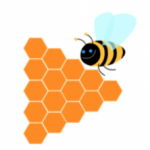Smartick is a fun way to learn math!# I want to learn about: Geometry

Learn elementary geometry with Smartick. Exercises, theory, tutorials, and teaching resources for children learning elementary geometry. Here you will find posts about geometry to make learning easier and more fun.

Aug26

## Geometric Shapes: Circle

We’ve already seen some of the main geometric shapes in earlier posts. In this post, we’re going to look more in-depth at the circle. A circle is a geometrical shape that is made by drawing a curve that always has the same distance from the point that we refer to as the center. In just […]

Jul11

## Geometric Figures and Straight LinesStraight lines are key to recognizing shapes that we often see in the real world: geometric figures. In today’s post, we will classify geometric figures formed from straight lines and think about where we can find them. We’ll start with the simplest: Two Straight LinesCan you make a figure with two straight lines? Well, of […]